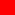## KALYAN CITY LIFE

Sharing Wisdom and Vivid Memories of Life

# International Units of Scientific Measurement - SI System

##International System of Units SI

Sevres, France based International Bureau of Weights and Measures, serves as a depository for the primary international standards and as a laboratory for certification and intercomparison of national standard copies. In 1960, the SI system was built upon the MKS system, that is, metre-kilogram-second system, which has seven basic units. These are as follows :-

Metre (unit of length; Symbol m) defined as the distance travelled by light in a vacuum in 1/299,792,458 second;

Kilogram (unit of weight; Symbol kg) which equals 1,000 grams as defined by the international prototype kilogram of platinum-iridium in the keeping of the International Bureau of Weights and Measures;

Second (unit of time; Symbol s), the duration of 9,192,631,770 periods of radiation associated with a specified transition of the cesium-133 atom;

Ampere (unit of electric current; Symbol A), which is the current that, if maintained in two wires placed one metre apart in a vacuum, would -produce a force of 2 x 10-7 Newton per metre of length;

Candela (unit of luminous intensity; Symbol cd), defined as the intensity in a given direction of a source emitting radiation of frequency 540 x 1012 hertz and that has a radiant intensity in that direction of 1/683 watt per steradian;

Mole (unit of amount of substance; Symbol mol), defined as containing as many elementary entities of a substance as there arc atoms in 0.012 kilogram of carbon-12

Kelvin (unit of temperature; Symbol K) which is 1/273.16 of the thermodynamic temperature of the triple point (equilibrium among the solid, liquid, and gaseous phases) of pure water.

##Units of Scientific Measurement

Atomic Weight : The weight of an atom of hydrogen is taken as the standard; the respective weights of the atoms of all other substances are expressed in terms of it. So when it is stated that the atomic weight of iron is 56, it is meant that the atom of iron is 56 times as the atom of hydrogen.

Angstrom : Unit of wavelength of light is Angstrom. 1 Angstrom = 10-8 cm. There is a bigger unit for measuring the wavelength of infra-red light; it is called a milli-micron and is equal to 10-7 cm. Micron = 10-4 cm, is a still bigger unit.

Bar : Unit of atmospheric pressure; one bar is equal to a pressure of 106 dynes per sq cm.

Calorie : Unit of heat. It is the amount of heat required to raise the temperature of one gram of water through 1°C.

Horse Power : The practical unit of power. The power of an agent which can work at the rate of 550 foot-pounds per second or 33,000 foot-pounds per minute. 1 HP = 746 watts

joule: Unit of work or energy. It is equal to 10-7 ergs. It is the energy consumed in one second in an electrical circuit through which a current energy of one ampere is flowing against a potential difference of one volt.

Knot : Measure to know the speed of a ship.

Light Year : A light year is the distance light travels in one mean 'solar year, at a speed of 1,86,000 miles per second. It is equal to 5,880,000,000,000 miles. It is used as a unit for measuring stellar distances.

Nautical Mile : A unit of distance used in navigation. One minute of longitude measured along the Equator. A Nautical Mile is approximately equal to 6,080 feet.

Pressure : The pressure is expressed in pounds weight per sq cm. The pressure of the atmosphere is expressed in millibars. One millibar = 1 dyne per sq cm. If the pressures are very high, they are expressed in multiples of atmospheric pressure. 1 atmosphere is a pressure exerted by a column of mercury 76 cm high at sea level and at a latitude of 45°.

Quintal : Metric measure of weight; 100 kilograms = 1 quintal.

1 ton = 10 quintal = 1000 kg.

Volt : The unit of potential difference. It is that much potential difference which when applied to the ends of an electrical conductor of resistance one ohm, the amount of energy consumed in the circuit in one second is one Joule (=107 ergs).